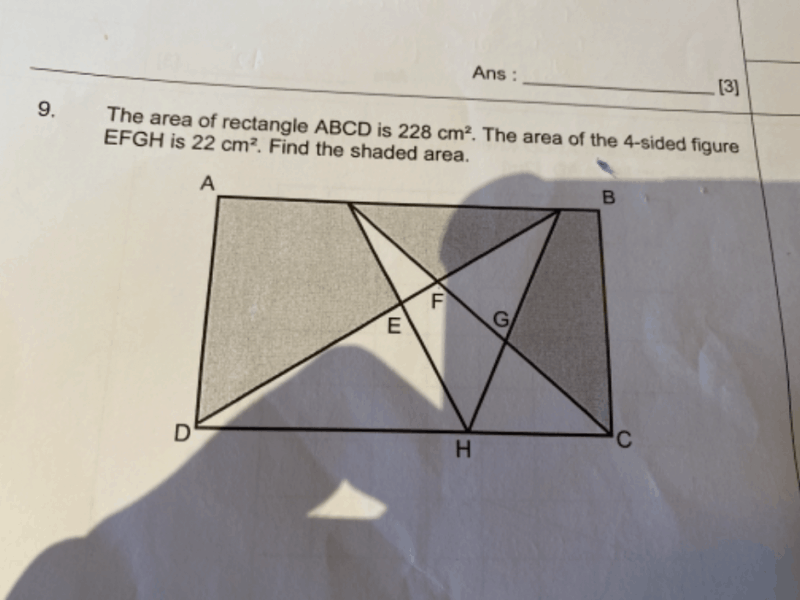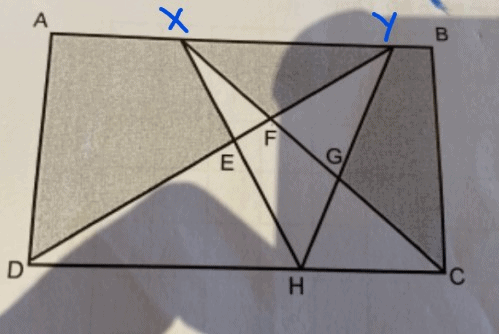# QuestionSource: Raffles Girls’ PrimaryHi Army, let me try to help you out with this question.

Before proceeding, I will add “x” and “y” on the figure so its easier to denote the shapes that I will be referring to.

The total area of DHY and HCX is actually equivalent to half of the rectangle ABCD. That means, the total area of these 2 triangles, when counted individually and then summed up together, will be 228/2 = 114cm2. There is an explanation as to why the total area of DHY and HCX is half of the area of ABCD, do contact me if you would like to hear about it (:

Knowing that the sum of the two triangles is 114cm2, we can see that there is an overlapped part, EFGH, which is 22cm2. We will subtract that part from 114cm2 to get 92cm2.

This means, our figure DEXFYGCH, has an area of 92cm2. To find the shaded area, subtract DEXFYGCH from ABCD, that will be 228cm– 92cm2 = 136cm2.

As mentioned, this question requires some background knowledge of how certain triangles will be half the area of the rectangles or squares. With this knowledge, students will also be able to better understand how the formula “half x base x height” is derived. I will be happy to share more with you, just let me know.

Hope this solution provided above helps!

Feel free to PM or text me (:

http://www.futureachievers.com.sg

9114 3397

0 Replies 2 Likes